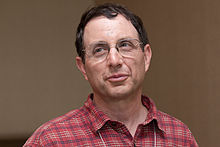# Neil Immerman

Neil ImmermanNeil Immerman in 2010.

Neil Immerman (24 November 1953, Manhasset, New York) is an American theoretical computer scientist, a professor of computer science at the University of Massachusetts Amherst. He is one of the key developers of descriptive complexity, an approach he is currently applying to research in model checking, database theory, and computational complexity theory.

Professor Immerman is an editor of the SIAM Journal on Computing and of Logical Methods in Computer Science. He received B.S. and M.S. degrees from Yale University in 1974 and his Ph.D. from Cornell University in 1980 under the supervision of Juris Hartmanis, another Turing award winner at Cornell. His book "Descriptive Complexity" appeared in 1999.

Immerman is the winner, jointly with Róbert Szelepcsényi, of the 1995 Gödel Prize in theoretical computer science for proof of what is known as Immerman–Szelepcsényi theorem, the result that nondeterministic space complexity classes are closed under complementation. Immerman is an ACM Fellow and a Guggenheim Fellow.

Wikimedia Foundation. 2010.

Поможем студентам написать доклад

### Look at other dictionaries:

• Neil Immerman — (2010) Neil Immerman (* 24. November 1953 in Manhasset, New York) ist ein amerikanischer Wissenschaftler im Bereich der theoretischen Informatik und Professor an der University of Massachusetts Amherst …   Deutsch Wikipedia

• Immerman–Szelepcsényi theorem — The Immerman–Szelepcsényi Theorem was proven independently by Neil Immerman and Róbert Szelepcsényi in 1987, for which they shared the 1995 Gödel Prize. In its general form the theorem states that NSPACE = co NSPACE. In other words, if a… …   Wikipedia

• St-connectivity — In computer science and computational complexity theory, st connectivity is a decision problem asking, for vertices s and t in a directed graph, if t is reachable from s .Formally, the decision problem is given by PATH = { | D is a directed graph …   Wikipedia

• Prix Gödel — Nommé en l honneur du logicien Kurt Gödel, le prix Gödel a été créé en 1992 par l European Association for Theoretical Computer Science (EATCS), l Association for Computing Machinery (ACM) et le groupe de l ACM sur l algorithmique et la théorie… …   Wikipédia en Français

• Descriptive complexity — is a branch of finite model theory, a subfield of computational complexity theory and mathematical logic, which seeks to characterize complexity classes by the type of logic needed to express the languages in them. For example, PH, the union of… …   Wikipedia

• BIT predicate — In mathematical logic, the BIT predicate, sometimes written BIT(i,j), is a predicate which tests whether the j th bit of the number i is 1, when i is written in binary. The BIT predicate is often examined in the context of first order logic,… …   Wikipedia

• Descriptive complexity theory — For other uses, see Kolmogorov complexity. Descriptive complexity is a branch of computational complexity theory and of finite model theory that characterizes complexity classes by the type of logic needed to express the languages in them. For… …   Wikipedia

• Automate linéairement borné — En informatique théorique, et en particulier en théorie des automates, un automate linéairement borné (en anglais linear bounded automaton, abrégé en LBA) est une machine de Turing non déterministe assujettie à des restrictions dans son mode… …   Wikipédia en Français

• First-order reduction — A first order reduction is a very weak type of reduction between two computational problems in computational complexity theory. A first order reduction is a reduction where each component is restricted to be in the class FO of problems calculable …   Wikipedia